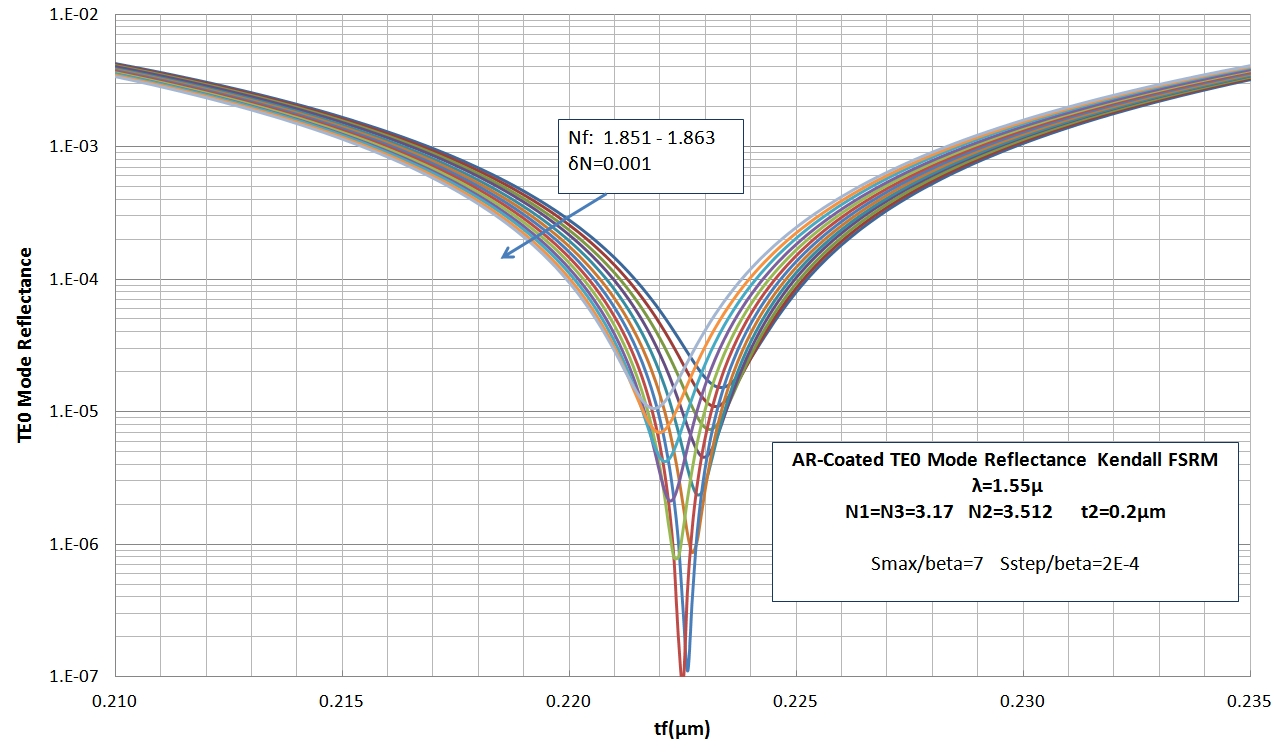## Wguide17M    Computational Convergence & Accuracy of Models

6/2017

The charts below provide some indication of computational precision and the accuracy of 3 approximate models. The first chart shows the shape of the Kendall FSRM reflectance integrand terms, for a single-layer AR coated waveguide, as a function of the integration parameter S/beta. Clearly the sharp structure in the numerator integration terms Num.Re and Num.Im, due mainly to discontinuities related to plane-wave component Fresnel external TIR reflectance effect relative to the external (air) medium, will determine the integration resolution required for sufficient numerical accuracy. The integrand denominator term Den.Re is essentially the same as the |FT(S)|^2 term and is essentially a field-normalization integral: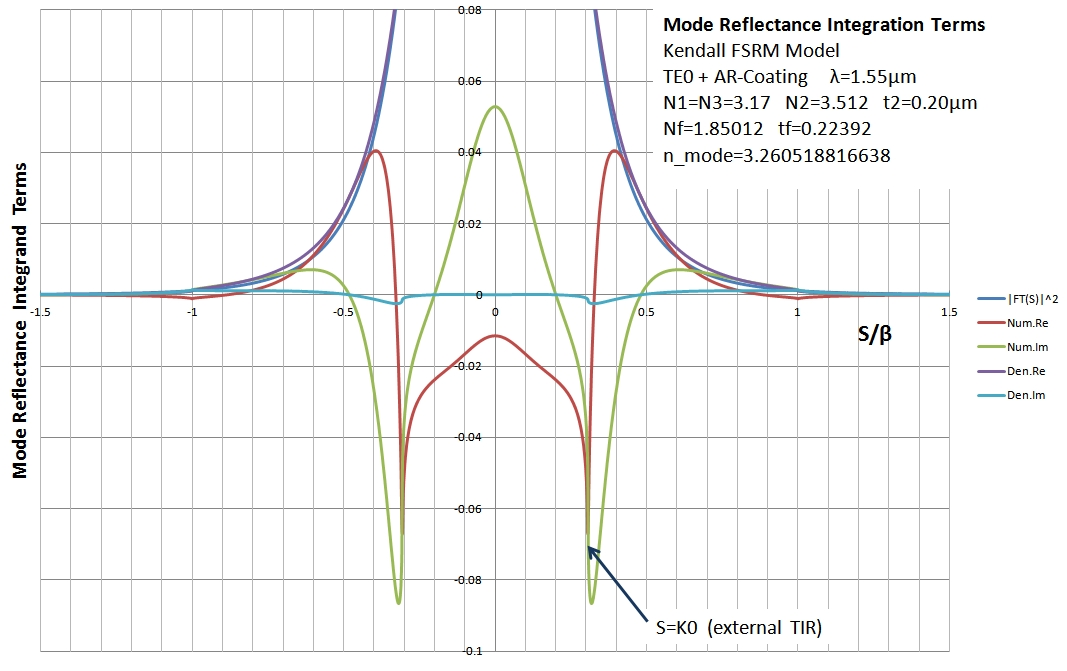The next 2 charts demonstrate computational convergence, for the same AR-coated waveguide as above, as a function of the normalized Fourier integration range parameter Smax/beta and the integration resolution Sstep/beta. Usually convergence to better than ~ 1% is achieved with Smax/beta > 5 and Sstep/beta < 0.001. The example below is for a fairly low reflectance single-layer antireflection coating using the Kendall FSRM model. Similar convergence applies to the other models and TM modes: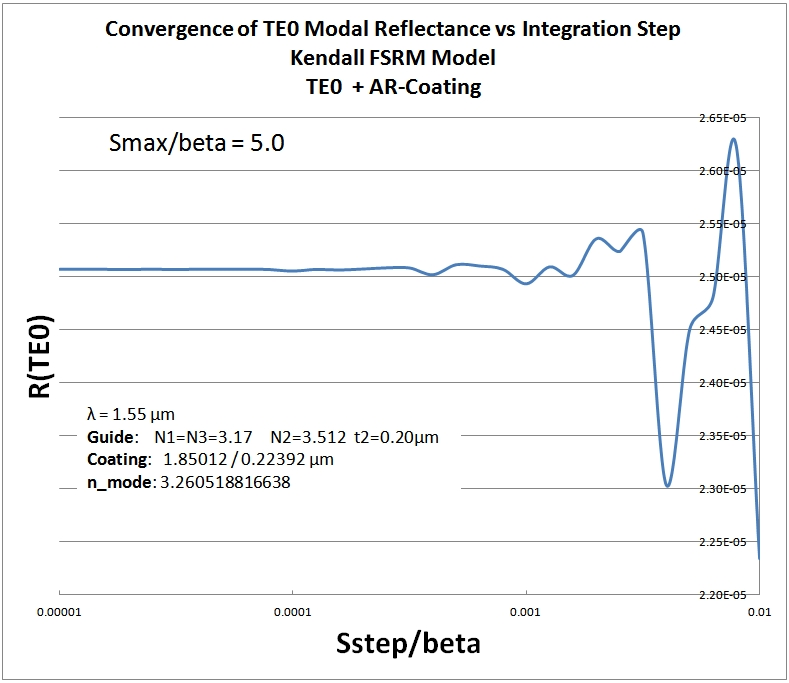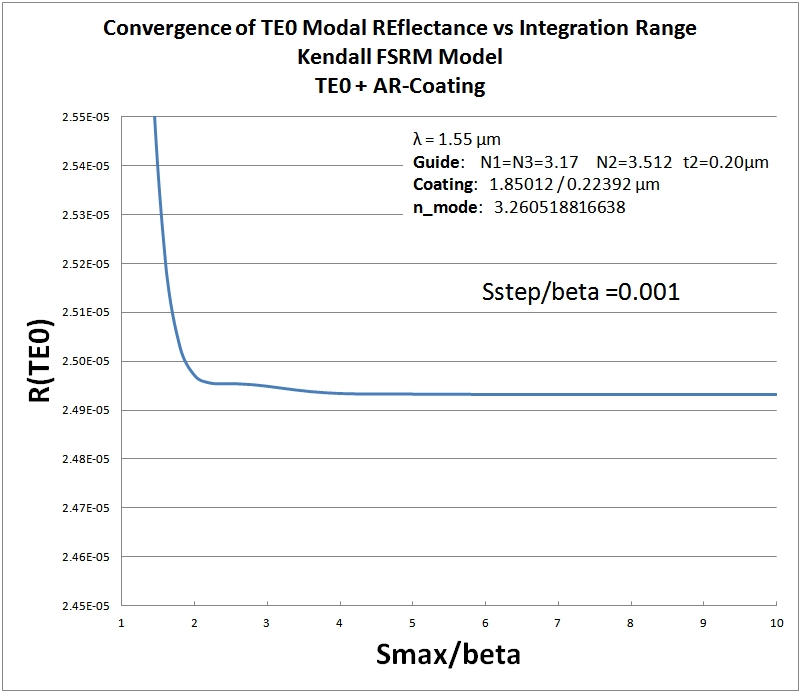The accuracy of the approximate models (how close the converged modal reflectance result is to the exact value as calculated by rigourous methods) is demonstrated below. Using the EXACT predicted coating index value (Vassallo 1988 p.1925) for a perfect (R< 1e-12) single layer AR coating design for the TE0 slab mode and for the waveguide configuration shown, the 3 curves demonstrate typical variation in the approximate results that can be expected. Both the Fresnel and the Vassallo88 results use the same "optimum" equivalent index neq in a fictitious layer plane wave calculation. The Kendall approach uses Free Space Radiation Modes with an approximate mode orthogonality enforced at the facet. From a design point of view, the variation in coating thickness predicted by the approximate models deviates by ~ 0.3% from the exact predicted value. By adjusting the coating layer refractive index very slightly, any of the approximate models will predict an extremely low R value of < 1e-10. The chart below provides some idea of the process control required on coating thickness and index to achieve R below a specific value. Using these approximate models to design coatings with reflectance values much below 1e-5 can be useful, but will not be very accurate, particularly when process control variations are considered and additionally 3 dimensional waveguide effects will likely be important in modifying very low R values from those predicted by ideal slab waveguide modelling: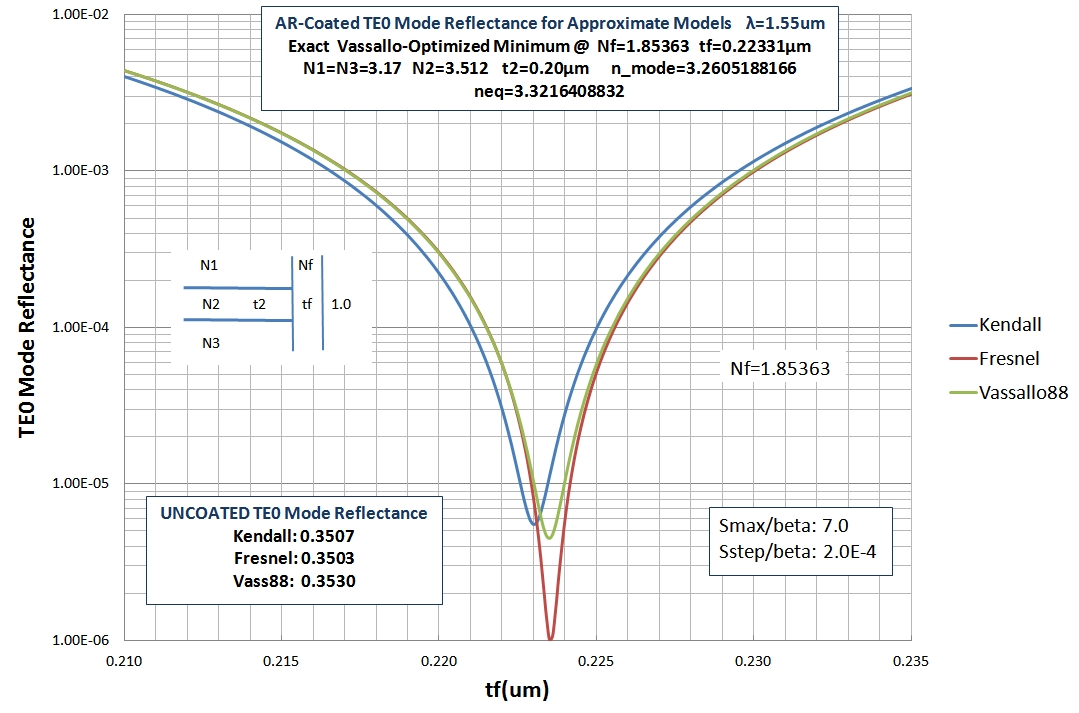As mentioned above, slight variation of single-layer coating index and thickness in the approximate models can produce almost perfect (<1e-10) reflectance values. The chart below for the same waveguide structure as the previous chart demonstrates this trend with the TE0 mode reflectance curves vs. coating thickness in the Kendall FSRM model with coating layer refractive index as curve parameter. Finer resolution plots near tf=0.2225um show R values below 1e-10: